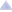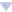### awoo's blog

By awoo, history, 3 weeks ago, translation,1832A - New Palindrome

Idea: BledDest

Tutorial
Solution (Neon)

1832B - Maximum Sum

Idea: BledDest

Tutorial
Solution (awoo)

1832C - Contrast Value

Idea: BledDest

Tutorial
Solution (Neon)

1832D1 - Red-Blue Operations (Easy Version)

Idea: BledDest

Tutorial

1832D2 - Red-Blue Operations (Hard Version)

Idea: BledDest

Tutorial
Solution (awoo)

1832E - Combinatorics Problem

Idea: BledDest

Tutorial
Solution (BledDest)

1832F - Zombies

Idea: BledDest

Tutorial
Solution (awoo)Comments (21)
 » imbalanceForces
 » F is so cool! Thanks for authors!
 » 3 weeks ago, # | ← Rev. 2 →   In the editorial of $E$, it is mentioned that $c_{i,0}=\sum_{j=1}^{i}{a_j}$. Actually, it should be $c_{i,0}=\sum_{j=1}^{i+1}{a_j}$ (with $c_{0,0}=a_1$ and $c_{n,0}$ is not needed).Reason:When we say $b_i$ (at $k$) $=$ $b_{i-1}$ (at $k$) $+$ $b_{i-1}$ (at $k-1$), this is true only when $k>1$, because this will cause the last term in both $\sum_{j=1}^{j=i}{{i-j \choose k} \cdot a_j}$ and $\sum_{j=1}^{j=i}{{i-j \choose k-1} \cdot a_j}$ to be $0$ (as $0 \choose x$ is $0$ for positive $x$). However, this is not the case for the $2^{nd}$ summation when $k=1$. The last term will not be eliminated as ${0 \choose 0}=1$.
•  » » https://codeforces.com/contest/1832/submission/205823199 I know it can be further optimised with prefix/suffix sum but where is my logic wrong in this code? can u plz explain....got same error during contest btw
•  » » » Take a look at Ticket 16856 from CF Stress for a counter example.
•  » » Fixed this issue, thank you!The editorial might take a while to update, but hopefully it will show the new version soon.
 » SorrowForces
 » 3 weeks ago, # | ← Rev. 3 →   My 3D Dp solution is giving wrong output ?? It would be huge help if someone explain . Thanks in advance !https://codeforces.com/contest/1832/submission/205945700
 » 3 weeks ago, # | ← Rev. 5 →   D1I see applying Operation 2 $n$ or $n-1$ times from begin.then, $k-(n-(n+k) ~\text{mod}~ 2)-1$ should be $k-(n-(n+k) ~\text{mod}~ 2)+1$ ?Or, $k-n+1 + (n+k) \text{mod}~2$
 » 3 weeks ago, # | ← Rev. 2 →   Problem B: I don't understand what is 'k — m' maximums, and I don't know what is k when m is the number of operations. Can anyone explain from me pls ??
•  » » 3 weeks ago, # ^ | ← Rev. 2 →   $k$ is given in the statement. upd: $k$ is the total number of operations, $m$ is the number of operations of the first type (when we delete two minimum elements)$(k-m)$ maximums is $(k-m)$ greatest elements of the array, i. e. $(k-m)$ last elements in sorted order.
 » I can't understand how he solved maximum sum. Can someone help me understand it?
•  » » The basic idea is that since you need to maximise the sum of the final array, you have to minimise the sum of the removed elements. So, first of all, we sort the array. Then we have to go through all the different combinations of selecting min and max elements. So, we will use a loop in which i will denote the number of starting elements (minimum elements) we will take (multiplied by two, since we have to delete two min elements at once) and k — i will be the number of elements from back (maximum elements). i = 0 means 0*2 = 0 elements from start and k -0 = k elements from end. i = 1 means 1*2 = 2 elements from start and k — 1 elements from end. .... i = k means k*2 elements from start and 0 elements from end.In each iteration, we need to get the sums of taking i*2 elements from start and k — i elements from the end. To get this sum in O(1) time we will use prefix sum.You can check my C++ code here https://codeforces.com/contest/1832/submission/205586590
•  » » » Oh i got the approach. Thanks a lot I will now try to code it.
 » For problem F, quadrangle inequality properties also apply to array partition DP. So we can use Knuth's optimization for a second time on $dp$, which leads an $O(n^2)$ algorithm.This is my submission: https://codeforces.com/contest/1832/submission/206185565.
 » Can problem A be solved in a different way other than unique function. Can't really understand it :(
 » 2 weeks ago, # | ← Rev. 2 →   Hi. can someone please tell me the error in this code which I used without prefix array #include #define endl "\n" #define int long long using namespace std; int maxRemoval(vector &v){ int n = v.size(), sum = 0; for(int i=0;i &v){ int n = v.size(), sum = 0; for(int i=2;i> t; while(t--){ int n, k; cin >> n >> k; vector v(n); for(int i=0;i> v[i]; } sort(begin(v), end(v)); int ans = 0; for(int i=0;i
•  » » I dont think that greede solution is correct. But it also O(n*k) and even it be correct, it gets TLE.
 » Why is this code getting wrong ans in TC 3 ?void solve() { int n;cin>>n; int k;cin>>k; vl a(n); fr(i,0,n) cin>>a[i]; sort(all(a)); `int l=0,r=n-k; ll sum=0ll; fr(i,0,r) sum+=a[i]; // cout<
 » For the C, there is a counter example of 5 2 5 3 7 9 10, which should give the output as 4 (7 9 3 10) but from the code it will give 5
 » 3 days ago, # |0.ComplaintFrame { display: inline-block; position: absolute; top: 0; right: -1.4em; } .ComplaintFrame a { text-decoration: none; color: #ff8c00; opacity: 0.5; } .ComplaintFrame a:hover { opacity: 1; } ._ComplaintFrame_popup p, ._ComplaintFrame_popup button { margin-top: 1rem; } ._ComplaintFrame_popup input[type=submit] { padding: 0.25rem 2rem; } ._ComplaintFrame_popup ul { margin-top: 1em !important; margin-bottom: 1em !important; } (first time commenting, apologize for bad formatting)Problem B:1 I try greedy by comparing oper1 and oper2, and it cant even pass example 5 cuz it gives me 12+13+15 = 40 instead of 10+11+12+13 = 46.2 I then try to do it in reversed order ie i assume that I do oper2 for k times first. ie remove maximum for kth time.https://codeforces.com/problemset/submission/1832/208181362then i compare the most recently deleted maximum and the sum of 2 minimums. restore the maximums and delete the 2 minimums by moving the ptrs, if the maximum is greater.It works most of the time.it fails when:9 350 51 60 61 62 63 102 103 900my code: 60+61+62+63+102 = 348answer: 102+103+900 = 11053 i change the code to do oper1 k times first. https://codeforces.com/problemset/submission/1832/208186847similar comparison of 2.it fails when:9 325 26 27 28 29 30 31 32 60my code: 31+32+60 = 123answer: 25+26+27+28+29+30 = 1654 i combine 2 and 3 by finding maximum of the results from two algorithms. https://codeforces.com/problemset/submission/1832/208192875then it works.Is there a reason that combining 2 and 3 give correct result for all of the cases?